## Filters

Sort by :
Clear All
Q
Engineering
121 Views   |

Which one of the following is the strongest base in aqueous solution?

• Option 1)

Methylamine

• Option 2)

Trimethylamine

• Option 3)

Aniline

• Option 4)

Dimethylamine

4

Engineering
110 Views   |

In the chemical reaction,   the compounds are respectively

• Option 1)

• Option 2)

• Option 3)

• Option 4)

As we learnt in carbylamnie Reaction - Product is isocyanide & this reaction is used for the detection of primary amines. - wherein                                                                                                Option 1) Correct Option 2) Incorrect Option 3) Incorrect Option 4) Incorrect
Engineering
152 Views   |

In the following sequence of reactions,

the compound is

• Option 1)

propanal

• Option 2)

butanal

• Option 3)

-butyl alcohol

• Option 4)

-propyl alcohol.

As we learnt in  Test for alcohols by Victor - Meyer's test - Reagents used  - wherein     Reaction of Haloalkanes with Mg in presence of ether - Grignard reagent is obtained     - wherein   Option 1) propanal This option is Incorrect Option 2) butanal This option is Incorrect Option 3) -butyl alcohol This option is Incorrect Option 4) -propyl alcohol. This option is Correct
Engineering
134 Views   |

The compound formed as a result of oxidation of ethyl benzene by is

• Option 1)

benzyl alcohol

• Option 2)

benzophenone

• Option 3)

acetophenone

• Option 4)

benzoic acid.

As we learnt in  Oxidation of Alkyl benzene - The entire side chain of alkyl benzene is oxidised to carboxyl group irrespective of the length of the side chain. - wherein     Option 1) benzyl alcohol This option is incorrect Option 2) benzophenone This option is incorrect Option 3) acetophenone This option is incorrect Option 4) benzoic acid. This option is correct
Engineering
110 Views   |

The secondary structure of a protein refers to

• Option 1)

fixed configuration of the polypeptide backbone

• Option 2)

• Option 3)

hydrophobic interactions

• Option 4)

sequence of

As we learnt in Secondary proteins -  configuration in which the polypeptide chain undergo into the tight coiling or folding - wherein It is of two types ,  helix and  sheet    Secondary structure of protein is of two types. d-helix and B-sheet. Option 1) fixed configuration of the polypeptide backbone This option is incorrect Option 2) This option is correct Option 3) hydrophobic...
Engineering
453 Views   |

The stability of dihalides of Si, Ge, Sn and Pb increases steadily in the sequence

• Option 1)

• Option 2)

• Option 3)

• Option 4)

As we learnt in  Oxidation state of carbon family - Exhibits O.S. of +4L and +2 (ionic) Ge, Sn and Pb +2 o.s. is prominent due to inert pair effect -    Due to inert pair effect, the stability of +2 oxidization state increases down the group. Option 1) This option is incorrect. Option 2) This option is incorrect. Option 3) This option is incorrect. Option 4) This option is correct.
Engineering
162 Views   |

Identify the incorrect statement among the following.

• Option 1)

reacts with hot and strong solution to give and

• Option 2)

Ozone reacts with   to give

• Option 3)

Silicon reacts with in the presence of air to give

• Option 4)

reacts with excess of to give .

As we learnt in Reaction of Cl with excess Ammonia - - wherein NH3 get oxidised by Cl2    8NH3+3Cl2 N2+6NH4Cl NH3 gets oxidized by Cl2.   Option 1)   reacts with hot and strong solution to give and This option is  incorrect. Option 2) Ozone reacts with   to give This option is  incorrect. Option 3) Silicon reacts with in the presence of air to give This option is  incorrect. Option...
Engineering
203 Views   |

Regular use of the following fertilizers increases the acidity of soil?

• Option 1)

Ammonium sulphate

• Option 2)

Potassium nitrate

• Option 3)

Urea

• Option 4)

Superphosphate of lime.

As we learnt in  Ammonium sulphate undergoes the following reaction This leads to acidity of soil     is a salt of a storng acid  and a weak base , on hydrolysis it produces ion   Option 1) Ammonium sulphate This answer is correct Option 2) Potassium nitrate This answer is incorrect Option 3) Urea This answer is incorrect Option 4) Superphosphate of lime. This answer is incorrect
Engineering
219 Views   |

The actinoids exhibit more number of oxidation states in general than the lanthanoids. This is because

• Option 1)

the orbitals extend further from the nucleus than the orbitals

• Option 2)

the orbitals are more buried than the orbitals

• Option 3)

there is a similarity between and  orbitals in their angular part of the wave function

• Option 4)

the actinoids are more reactive than the lanthanoids.

As we learnt in Oxidation states - The common oxidation state of lanthanoid is +3 low occasionally +2  and  +4  ions in solution or in the solid compound are also obtained. -    and Oxidation states - Ac shows +3 oxidation state generally but some Ac also, stable till +7 oxidation states. -   Actinoids exhibits more number of exidation states in general than the Lanthanoids. This is because the...
Engineering
91 Views   |

The equivalent conductances of two strong electrolytes at infinite dilution in ( where ions move freely through a solution ) at 25°C are given below:

What additional information/quantity one needs to calculate of an aqueous solution of acetic acid?

• Option 1)

of chloroacetic acid

• Option 2)

• Option 3)

• Option 4)

The limiting equivalent conductance of

As we learnt in Application of Kohlrausch's law - Calculation of molar conductivities of weak electrolyte at infinite dilution. -     According to Kohlrausch’s law, the molar conductivity at infinite dilution for weak electrolyte, So, for calculating the value of  , value  of should also be known. Option 1) of chloroacetic acid This option is incorrect. Option 2) This option is...
Engineering
130 Views   |

The cell,

was allowed to be completely discharged at 298 K .The relative concentration of

is

• Option 1)

• Option 2)

• Option 3)

• Option 4)

As we learnt in Electrode Potential(Nerst Equation) - - wherein is concentration of species F= 96487 C  R= 8.314  T= Temperature in kelvin      When the cell is completely discharged, Option 1) This option is incorrect. Option 2) This option is incorrect. Option 3) This option is incorrect. Option 4) This option is correct.
Engineering
170 Views   |

Consider the reaction, products. When concentration of alone was doubled, the half­-life did not change. When the concentration of alone was doubled, the rate increased by two times. The unit of rate constant for this reaction is

• Option 1)

• Option 2)

• Option 3)

• Option 4)

As we learnt in  Rate of Law = Dependence of Rate on Concentration - The representation of rate of a reaction in terms of concentration of the reactants is known as Rate Law or The Rate Law is the expression in which reaction rate is given in terms of molar concentration of reactants with each term raised to some power, which may/maynot be equal to stoichiometric of the reacting species in a...
Engineering
154 Views   |

The energies of activation for forward and reverse reactions for respectively. The presence of a catalyst lowers the activation energy of both (forward and reverse) reactions by . The enthalpy change of the reaction

in the presence of a catalyst will be

• Option 1)

20

• Option 2)

300

• Option 3)

120

• Option 4)

280

As we learnt in  Catalyst - A catalyst is a surface that increases the rate of a chemical reaction without itself being used up. It remains unchanged chemically providing an alternate path for the reaction at an accelerated rate by lowering the activation energy.  - wherein    Enthalpy change of reaction do not change by adding catalyst.   Option 1) 20 This option is correct Option...
Engineering
133 Views   |

In conversion of limestone to lime,

the values of are +179.1 kJ mol-1 and 160.2 J/K respectively at 298 K and 1 bar. Assuming that do not change with temperature, temperature above which conversion of limestone to lime will be spontaneous is

• Option 1)

1118 K

• Option 2)

1008 K

• Option 3)

1200 K

• Option 4)

845 K

As we learnt in Entropy for phase transition at constant pressure - - wherein Transition Fusion, Vaporisition, Sublimation Enthalpy Internal Energy Transitional temperature     Option 1) 1118 K This option is correct. Option 2) 1008 K This option is incorrect. Option 3) 1200 K This option is incorrect. Option 4) 845 K This option is incorrect.
Engineering
103 Views   |

Assuming that water vapour is an ideal gas, the internal energy change when 1 mol of water is vapourised at 1 bar pressure and 1000C, (given : molar enthalpy of vapourisation of water at 1 bar and 373 K = 41 kJ mol-1 and R = 8.3 J mol-1 K-1) will be

• Option 1)

41.00 kJ mol-1

• Option 2)

4.100 kJ mol-1

• Option 3)

3.7904 kJ mol-1

• Option 4)

37.904 kJ mol-1

As we learnt in Molar heat capacity for isobaric process C(p) -   - wherein or    Given for n = 1 Option 1) 41.00 kJ mol-1 This option is incorrect. Option 2) 4.100 kJ mol-1 This option is incorrect. Option 3) 3.7904 kJ mol-1 This option is incorrect. Option 4) 37.904 kJ mol-1 This option is correct.
Engineering
347 Views   |

Identify the correct statement regarding a spontaneous process:

• Option 1)

Lowering of energy in the reaction process is the only criterion for spontaneity.

• Option 2)

For a spontaneous process in an isolated system, the change in entropy is positive.

• Option 3)

Endothermic processes are never spontaneous.

• Option 4)

Exothermic processes are always spontaneous.

As we learnt in Spontanous process -   - wherein For spontanouse process must be negative.    Spontaneity of reaction depends on tendency to acquire minimum energy state and maximum randomness. For a spontaneous process in an isolated system, the change in entropy is positive. Option 1) Lowering of energy in the reaction process is the only criterion for spontaneity. This option is...
Engineering
338 Views   |

Which of the following reactions will yield 2,2­-dibromopropane?

• Option 1)

• Option 2)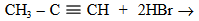• Option 3)

• Option 4)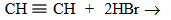As we learnt in  Chemical Reaction Halogenation of alkane - When alkane react with halogen in presence of UV light it give free radical substitution reaction. - wherein So correct option is 2.   Option 1) This option is incorrect. Option 2) This option is correct. Option 3) This option is incorrect. Option 4) This option is incorrect.
Engineering
281 Views   |

Which of the following hydrogen bonds is the strongest?

• Option 1)

• Option 2)

• Option 3)

• Option 4)

As we learnt in

Hydrogen Bonding -

Attractive force which binds hydrogen atom of one molecule with the electronegative ( F, O, N ) atom of another molecule.

- wherein

It is of two type

1.  Intermolecular and

2.  Intramolecular

Because of highest electronegativity of F, hydrogen bonding in Is strongest.

Option 1)

This option is incorrect

Option 2)

This option is incorrect

Option 3)

This option is correct

Option 4)

This option is incorrect

View More
Engineering
325 Views   |

In which of the following ionization processes, the bond order has increased and the magnetic behaviour has changed?

• Option 1)

• Option 2)

• Option 3)

• Option 4)

As we learnt in

Bond Order -

Bond order is defined as one half the difference between the number of electrons present in the bonding and the antibonding orbitals.

- wherein

and

Magnetic behaviour of molecule -

If  all the molecular orbitals in a molecule are doubly occupied, the substance is diamagnetic

- wherein

However if one or more molecular orbital are singly occupied it is paramagnetic e.g. O2

Molecular orbital configuration of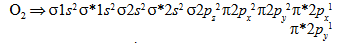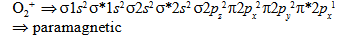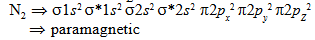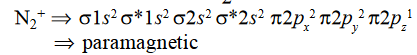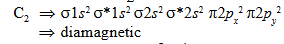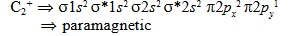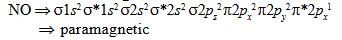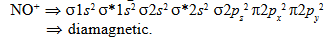Option 1)

This option is incorrect

Option 2)

This option is incorrect

Option 3)

This option is correct

Option 4)

This option is incorrect

View More
Engineering
131 Views   |

The charge/size ratio of a cation determines its polarizing power. Which one of the following sequences represents the increasing order of the polarizing power of the cationic species,

• Option 1)

• Option 2)

• Option 3)

• Option 4)

As we learnt in

Fajans Rules -

It accounts  for the covalent character in ionic compounds covalency is favoured by

1.  Small cation

2.  Large anion and

3.  Large charge on either ion

-

High charge and small size of the cations increases polarisation.

As the size of given cations decreases, polarisation power of cation increases. In order of polarisation power is

Option 1)

This option is incorrect

Option 2)

This option is incorrect

Option 3)

This option is incorrect

Option 4)

This option is correct

View More
Exams
Articles
Questions